# IB DP Physics: SL复习笔记2.4.1 Force & Momentum

### Force & Momentum

• Linear momentum, p, is defined as the product of mass and velocity• Momentum is a vector quantity - it has both a magnitude and a direction
• This means it can have a negative or a positive value
• If an object travelling to the right has positive momentum, an object travelling to the left (in the opposite direction) has a negative momentum
• The negative or positive directions are defined by the observer on a case-by-case basis
• The SI unit for momentum is kg m s−1

#### Direction of Momentum

• If a ball of mass 60 g travels at 2 m s−1, it will have a momentum of 0.12 kg m s−1
• If it then hits a wall and rebounds in the exact opposite direction, it will have a momentum of −0.12 kg m s−1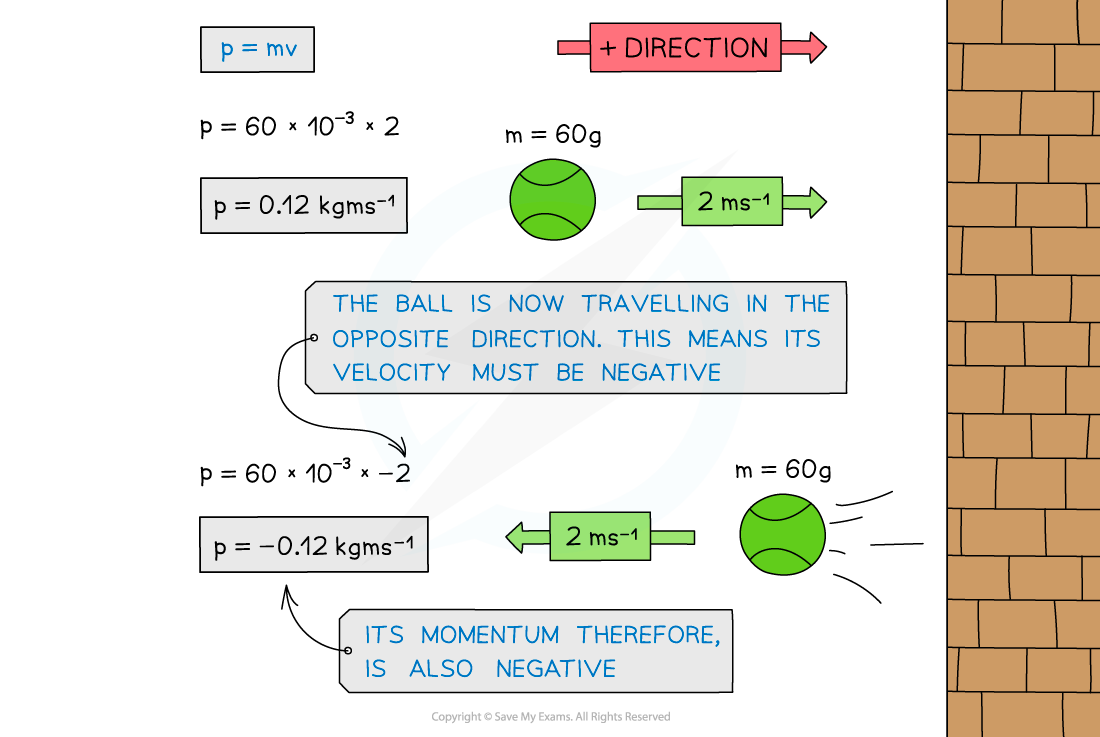When the ball is travelling in the opposite direction, its velocity is negative. Since momentum = mass × velocity, its momentum is also negative

#### Worked Example

Determine which object has the most momentum.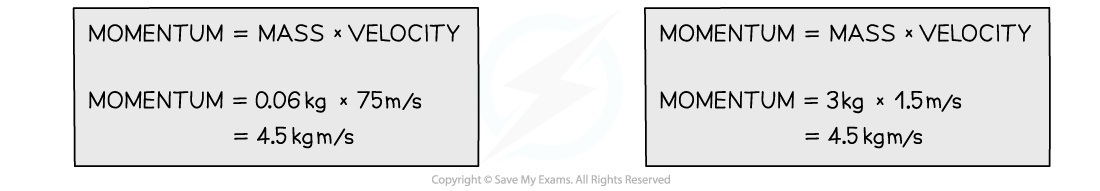• Both the tennis ball and the brick have the same momentum
• Even though the brick is much heavier than the ball, the ball is travelling much faster than the brick
• This means that on impact, they would both exert a similar force (depending on the time it takes for each to come to rest)

#### Force & Momentum

• Force is defined as the rate of change of momentum on a body
• The change in momentum is defined as the final momentum minus the initial momentum
• These can be expressed as follows: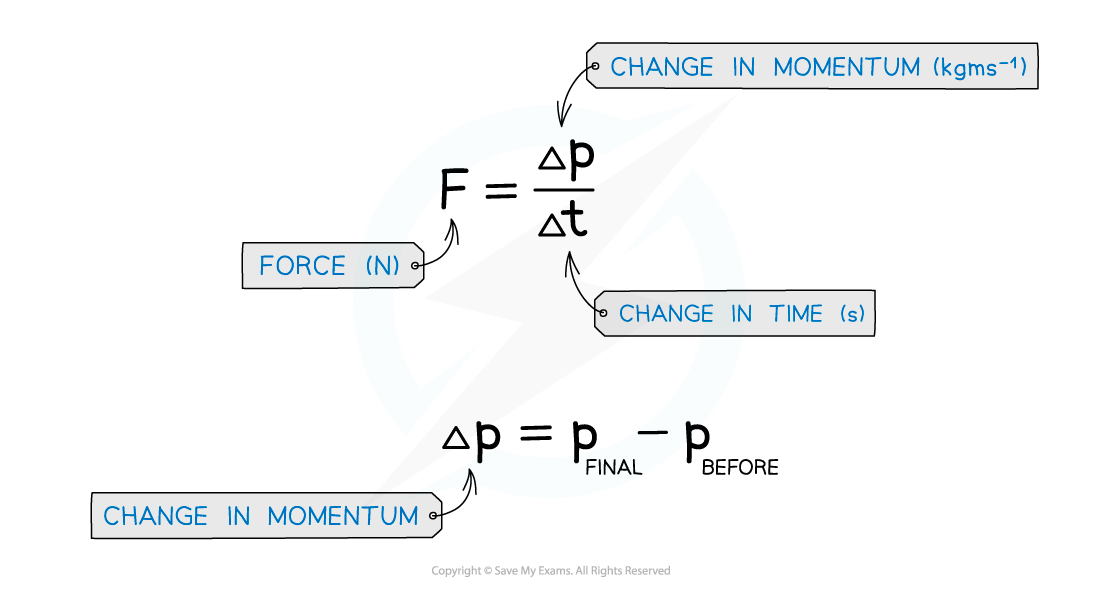• It should be noted that the force in this situation is equivalent to Newton's second law:

F = m × a

• Only when mass is constant
• In situations where mass is not constant, Newton's second law can only be considered to assist descriptions and not for calculations
• The force and momentum equation can be derived from Newton's Second law and the definition of acceleration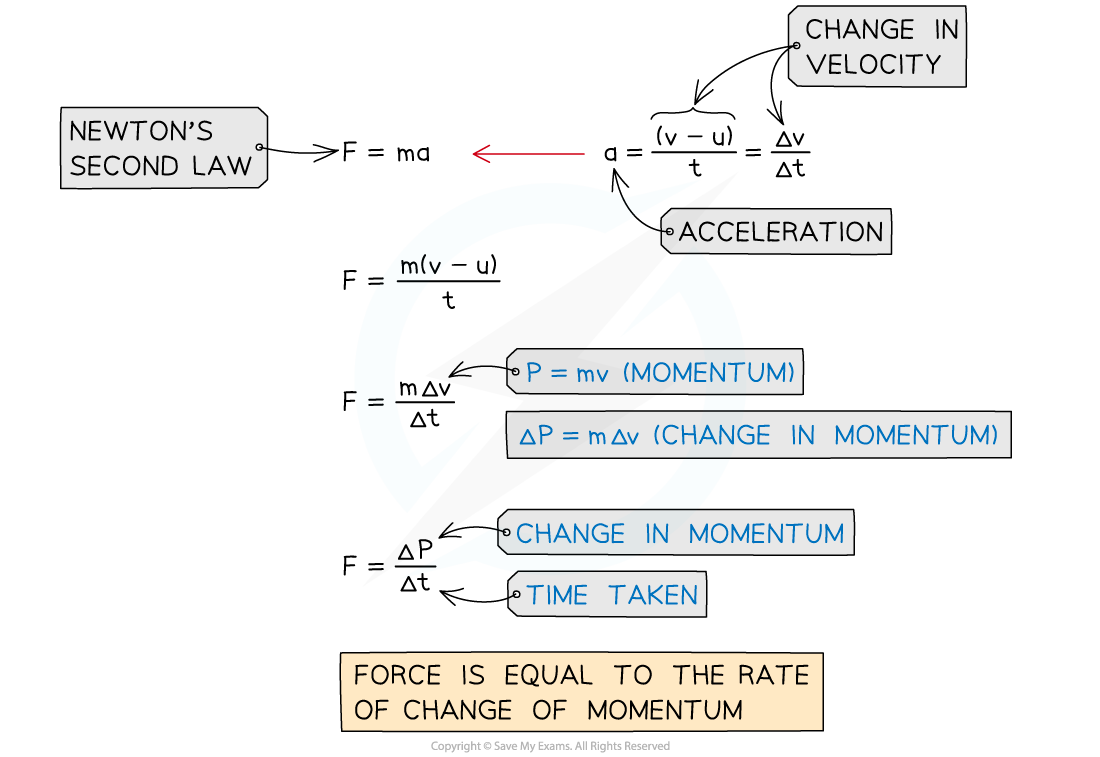#### Direction of Forces

• Force and momentum are vectors so they can take either positive or negative values
• The force that is equal to the rate of change of momentum is still the resultant force
• A force on an object will be negative if it is directed in the opposite motion to its initial velocity
• This means that the force is produced by the object it has collided with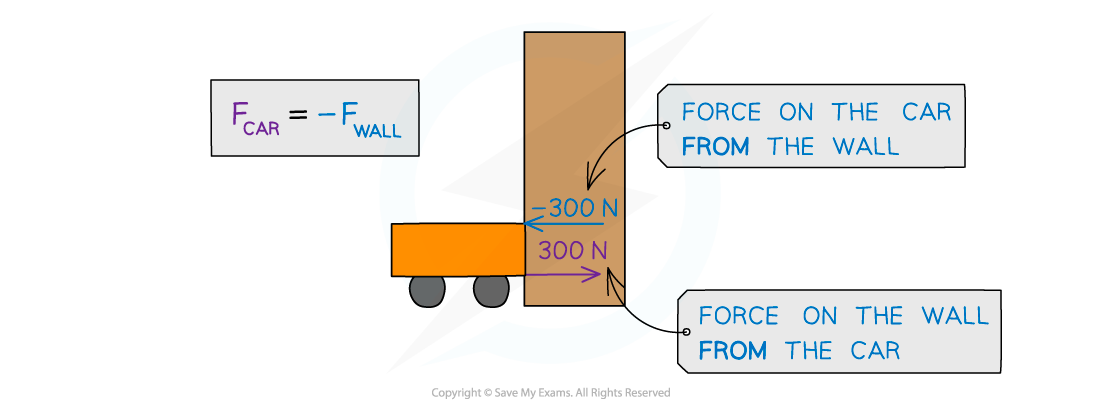The wall produces a force of -300N on the car and (due to Newton’s Third Law) the car also produces a force of 300 N back onto the wall

#### Worked Example

A car of mass 1500 kg hits a wall at an initial velocity of 15 m s−1. It then rebounds off the wall at 5 m s−1 and comes to rest after 3.0 s.Calculate the average force experienced by the car.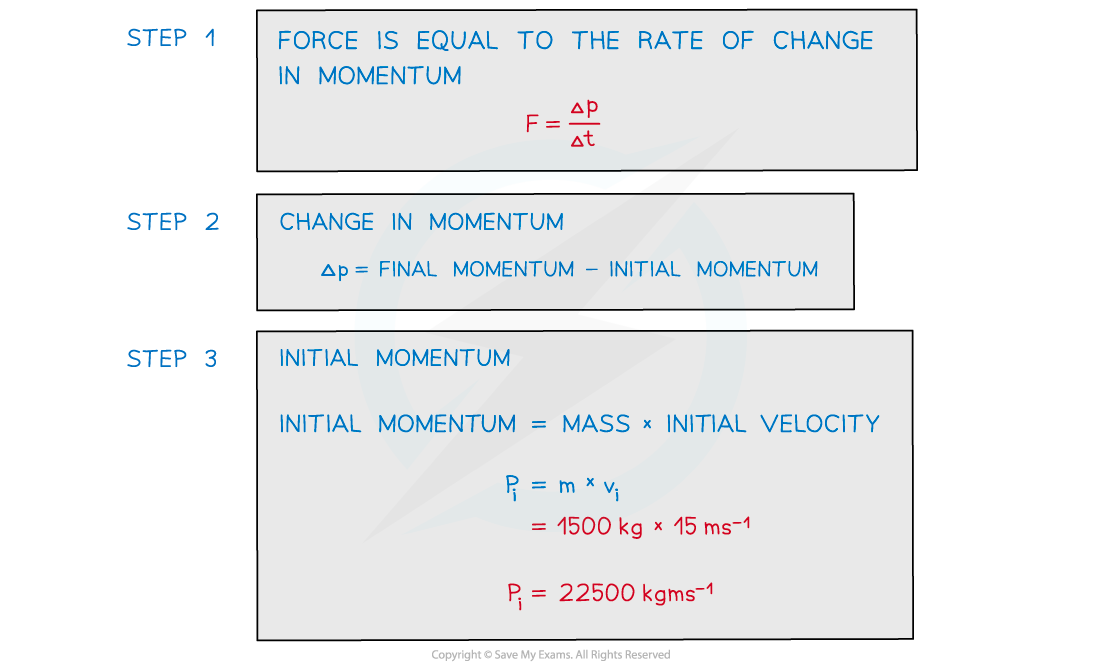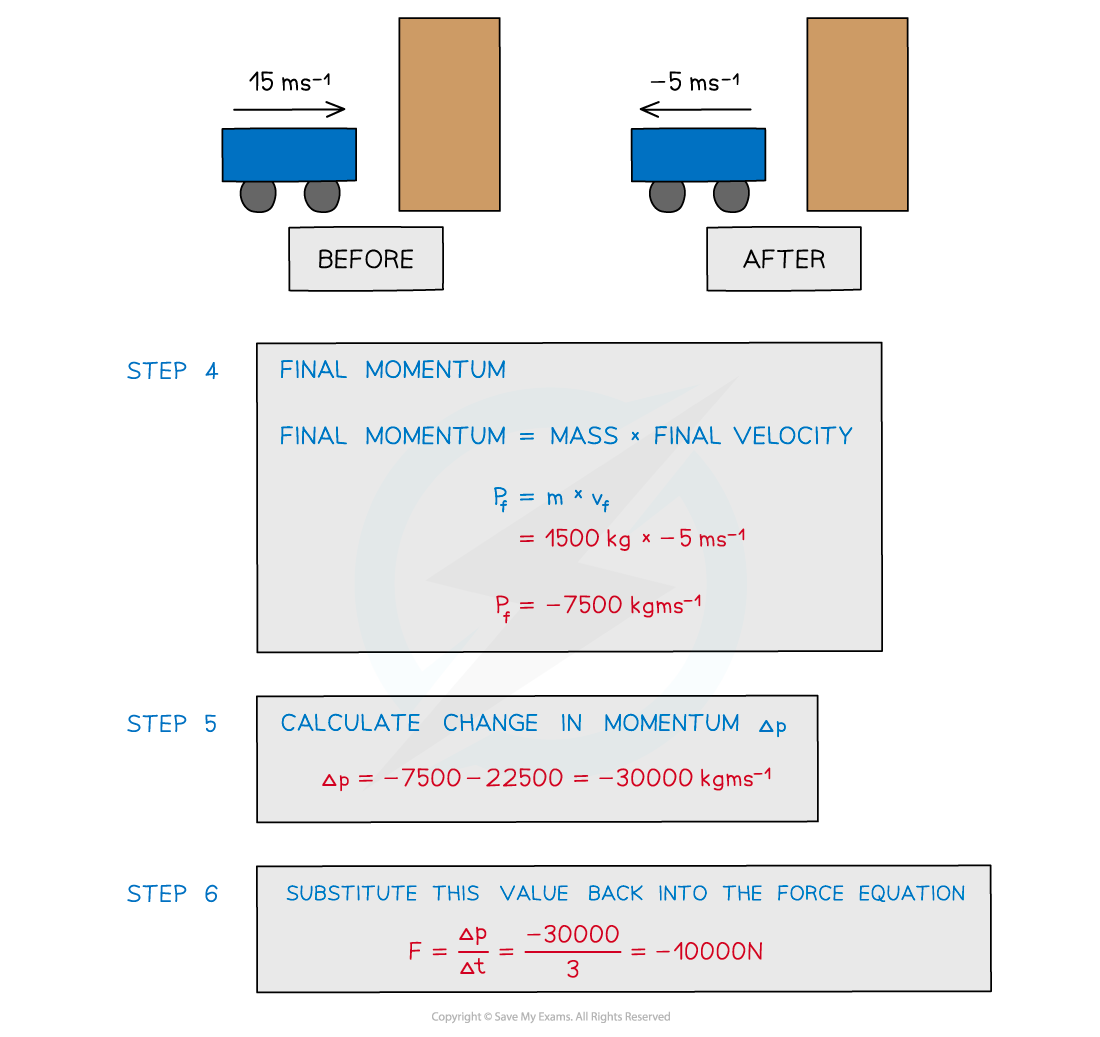#### Exam Tip

The direction you consider positive is your choice, as long the signs of the numbers (positive or negative) are consistent with this throughout the questionIn an exam question, carefully consider what produces the force(s) acting. Look out for words such as ‘from’ or ‘acting on’ to determine this and don’t be afraid to draw a force diagram to figure out what is going on.